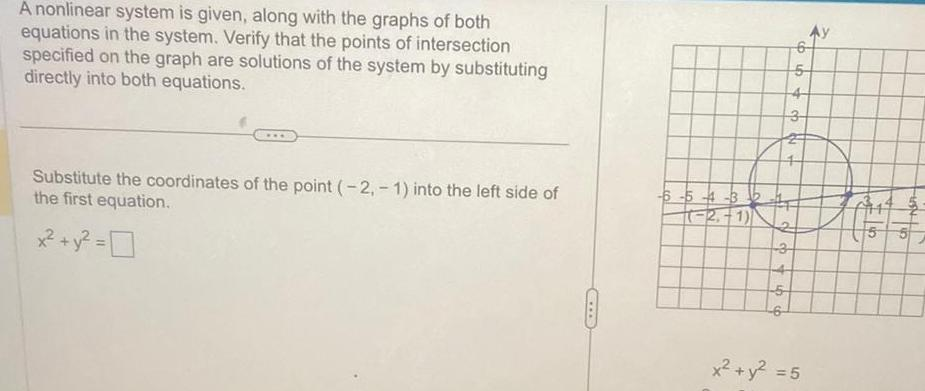Question:

# A nonlinear system is given along with the graphs of both

Last updated: 11/21/2023A nonlinear system is given along with the graphs of both equations in the system Verify that the points of intersection specified on the graph are solutions of the system by substituting directly into both equations Substitute the coordinates of the point 2 1 into the left side of the first equation x y 0 6 5 4 3 2 1 4 3 3 6 5 5 x y 5 116